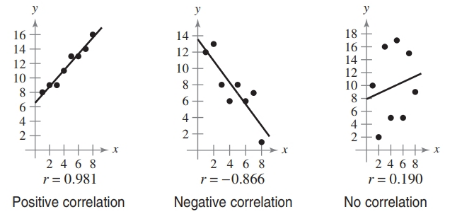Chapter 7.7, Problem 22E### Calculus: An Applied Approach (Min...

10th Edition
Ron Larson
ISBN: 9781305860919

#### Solutions

Chapter
Section### Calculus: An Applied Approach (Min...

10th Edition
Ron Larson
ISBN: 9781305860919
Textbook Problem
1 views

# Determining Correlation In Exercises 19–24, plot the points and determine whether the data have positive, negative, or no linear correlation (see figures below). Then use a graphing utility to find the value of r and confirm your result. The number r is called the correlation coefficient. It is a measure of how well the model fits the data. Correlation coefficients vary between −1 and 1, and the closer ∣r∣ is to 1, the better the model.(1, 36), (2, 10), (3, 0), (4, 4), (5, 16), (6, 36)

To determine

To graph: The points (1,36),(2,10),(3,0),(4,4),(5,16),(6,36) and determine whether these points have positive, negative or no linear correlation. Also determine the correlation coefficient using graphing utility.

Explanation

Given Information:

The points (1,36),(2,10),(3,0),(4,4),(5,16),(6,36).

Graph:

Consider the provided point (1,36),(2,10),(3,0),(4,4),(5,16),(6,36).

The graph (1,36),(2,10),(3,0),(4,4),(5,16),(6,36)

Therefore, the data points have no correlation with r=0.075.

To determine the correlation coefficient using TI-83 calculator follow the steps given below,

Step 1: Press STAT key and then press 1 to select Edit.

Step 2: Enter the provided coordinates as,

 L1 L2 1 36 2 10 3 0 4 4 5 16 6 36

Step 3: Press 2nd and then 0 key to enter into CATALOG section

### Still sussing out bartleby?

Check out a sample textbook solution.

See a sample solution

#### The Solution to Your Study Problems

Bartleby provides explanations to thousands of textbook problems written by our experts, many with advanced degrees!

Get Started

#### Compute P9,9.

Understanding Basic Statistics

#### In Problems 7-34, perform the indicated operations and simplify. 33.

Mathematical Applications for the Management, Life, and Social Sciences

#### Sometimes, Always, or Never: If 0 ≤ an ≤ bn for all n and diverges, then converges.

Study Guide for Stewart's Single Variable Calculus: Early Transcendentals, 8th